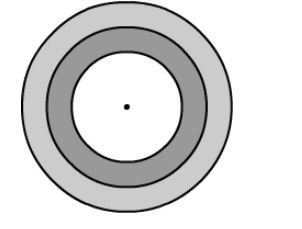# If the diameters of the concentric circles shown in the figure below are in the ratio 1 : 2 : 3`
Question:

If the diameters of the concentric circles shown in the figure below are in the ratio 1 : 2 : 3 then find the ratio of the areas of three regions.Solution:

The diameters of concentric circles are in ratio 1 : 2 : 3.

let the diameters be $d_{1}=1 x_{1} d_{2}=2 x_{1} d_{3}=3 x$.

So, the radii are $r_{1}=\frac{x}{2}, r_{2}=x, r_{3}=\frac{3 x}{2}$.

The areas of three regions are given be

$A_{1}=\pi r_{1}^{2}=\pi\left(\frac{x}{2}\right)^{2}=\frac{\pi x^{2}}{4}$

$\mathrm{~A}_{2}=\pi r_{2}^{2}-\pi r_{1}^{2}=\pi x^{2}-\frac{\pi x^{2}}{4}=\frac{3 \pi x^{2}}{4}$

$\mathrm{~A}_{3}=\pi r_{3}^{2}-\pi r_{2}^{2}=\pi\left(\frac{3 x}{2}\right)^{2}-\pi x^{2}=\frac{5 \pi x^{2}}{4}$

So, their ratio is $A_{1}: A_{2}: A_{3}=\frac{\pi x^{2}}{4}: \frac{3 \pi x^{2}}{4}: \frac{5 \pi x^{2}}{4}=1: 3: 5$.